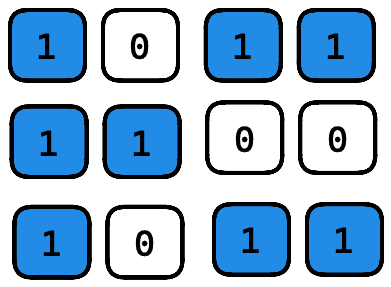# 0 1 MatrixLeetcode 542. 01 Matrix

##### Problem

Given an `m x n` binary matrix `mat`, return the distance of the nearest `0` for each cell.

The distance between two adjacent cells is `1`.

Example 1:

```Input: mat = [[0,0,0],[0,1,0],[0,0,0]]
Output: [[0,0,0],[0,1,0],[0,0,0]]
```

Example 2:

```Input: mat = [[0,0,0],[0,1,0],[1,1,1]]
Output: [[0,0,0],[0,1,0],[1,2,1]]
```

Constraints:

• `m == mat.length`
• `n == mat[i].length`
• `1 <= m, n <= 104`
• `1 <= m * n <= 104`
• `mat[i][j]` is either `0` or `1`.
• There is at least one `0` in `mat`.

Source: Leetcode 542. 01 Matrix

##### Solution

This is a classic BFS problem. We can measure distance from each 0 cell to 1 cells. To do that, we can push all the 0s in a queue and while the queue is not empty, we can expand search. If there is a 1 cell, we can update the distance of that cell with its parent cell’s distance plus 1.

Below code is self explanatory to understand the solution.

###### C++ Code
```class Solution {
public:
vector<vector<int>> updateMatrix(vector<vector<int>>& mat) {
int rows = mat.size();
if(rows==0) return mat;

int cols = mat.size();
queue< pair<int,int> > q; // Queue to maintain BFS
vector<vector<int>> dist(rows, vector<int>(cols, INT_MAX)); // distance vector, initialized with very high number

for(int i=0; i<rows; i++)
for(int j=0; j<cols; j++)
if(mat[i][j]==0){
q.push({ i , j });
dist[i][j] = 0; //distance of 0 cell is 0
}

int direction = { {-1,0}, {1,0}, {0,-1}, {0,1} };

while(!q.empty()){
pair<int,int> cur = q.front(); //take the current cell
q.pop();
int cur_r = cur.first;
int cur_c = cur.second;

for(int i=0; i<4; i++){ //explore into 4 directions
int  next_r = cur_r + direction[i];
int  next_c = cur_c + direction[i];

if(next_r >= 0 && next_c >= 0 && next_r < rows && next_c < cols){
/*
Here is the trick. Remember, we only made 0 cells distance = 0.
That means, any other cell that has INT_MAX distance is a cell
with 1. So, we will simply update it's distance with its parent
distance + 1.
*/
if( dist[next_r][next_c] > dist[cur_r][cur_c] + 1){
dist[next_r][next_c] = dist[cur_r][cur_c] + 1;
q.push({next_r, next_c});
}
}
}
}

return dist;
}
};```